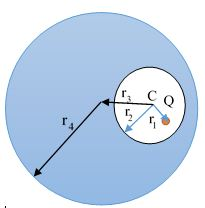# Electric potential due to conductors and cavities

Inside a neutral spherical conductor, a spherical cavity is present. A charge Q is placed inside the cavity. What is the electric potential at the center (C) of the cavity?In the diagram above,

• $r_1$ is the distance of the charge from the cavity's center C;
• $r_2$ is the radius of the cavity;
• $r_3$ is the distance between the centers of the sphere and cavity;
• $r_4$ is the radius of the sphere.
×